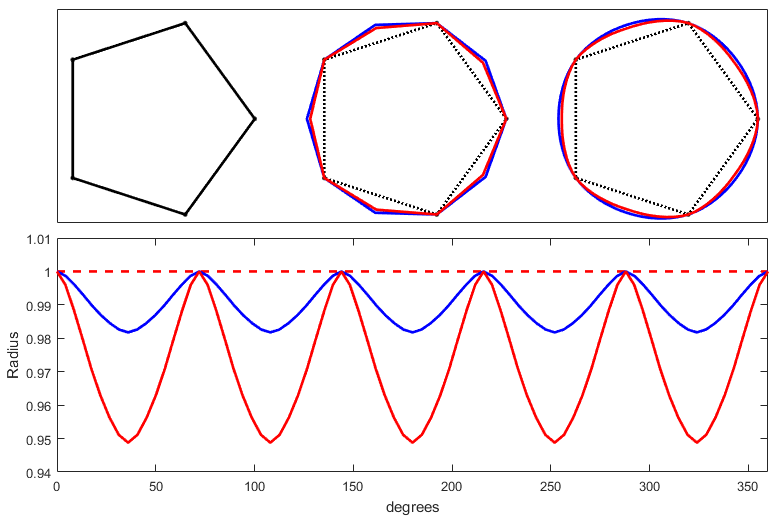Categories

# Chaikin-like Smoothing, Through Vertices

The standard Chaikin smoothing is a corner-cutting algorithm, which leads to nice results, but it no longer passes through the vertices of the original path. In cases where we trust the vertices more than the segments, what are we to do? Well, invent a new method, of course.

Ideally, I want to give this method a regular n-gon and receive a circle after repeated iterations. So after one iteration, it should output (very nearly) a 2*n-gon. Hmm. That means that I need to push out the midpoint of the segment. Let’s say that there is some angle at each endpoint of the vertex, project some points along those angles, and take the average. Pictures help:

Let’s concentrate on side $\overline{BC}$, and try to determine the position of the new point, $N=B'+C'$. But how should we define those intermediate points? The first way is to have them project parallel to the neighboring sides with some scale factor $s$:

\begin{aligned} B_1' &= B+ s _1 \, |\overline{BC}| \frac{B-A}{| \overline{AB}| } \\ C _1 ' &= C+ s _1 |\overline{BC}| \frac{C-D}{| \overline{DC}| } \end{aligned}

The second way is to project them with an angle that is tangent to the vertex:

\begin{aligned} B_2' &= B+ s _2 \, |\overline{BC}| \frac{C-A}{| \overline{AC}| } \\ C _2 ' &= C+ s _2 |\overline{BC}| \frac{B-D}{| \overline{BD}| } \end{aligned}

In either case, $N_x = 0$, and we want $N_y = 1$ (assuming a unit-radius n-gon). Solving for the scale factor yields:

\begin{aligned} s_1 &= \frac{1}{8} \sec \left(\frac{\pi}{2n} \right)^2 \sec \left(\frac{\pi}{n} \right) \\ s_2 &= \frac{1}{4} \sec \left(\frac{\pi}{2n} \right)^2 \end{aligned}

In general, we won’t know the number of sides, so let’s say $s_1 \approx 1/8, \, s_2 \approx 1/4$ in general. It turns out that the second version gives a slightly better approximation. Have a look at a comparison:Method 1 is in red, method 2 is in blue. The top shows one iteration, and 4 iterations. The bottom shows the radius of the approximations.

You can see that there’s minimal visual difference between the two when applied to a regular n-gon, but that method 2 is slightly better at making a circle. What about applying this to a more realistic path?

Eh, they both work fine.

After making all this, I finally figured out the right keywords to search for. This is a type of “outer smoothing” method, since it makes paths around the outside of a closed polygon, rather than in the inside as with Chaikin’s method.

This site uses Akismet to reduce spam. Learn how your comment data is processed.